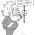### Is Atmospheric CO2 Increasing Exponentially?

I saw someone ask this on reddit and was surprised that no clear breakdowns showed up when I googled so I figured I'd work it out...

#### What is an exponential increase?

Exponential growth is most simply stated as growth proportional to the current value. For example, consider the function y = 2^t. When t = 1, y = 2. When t = 2, y = 4. When t = 3, y = 8. When t = 4, y = 16. Now...y(2)/y(1) = 2/1 = 2. y(3)/y(2) = 4/2 = 2. In general, y(t + 1)/y(t) = 2. You get a constant when dividing consecutive terms.

Looking at the rates, consider the difference instead of the ratios. y(2) - y(1) = 1. y(3) - y(2) = 2. y(4) - y(3) = 4. The rate is increasing. Is the increase related to the current value? Two easy ways to see it are to put it in a table and to plot it. First, the table:

tydiffdiff/value
12N/AN/A
2420.5
3840.5
41680.5

Notice how the difference divided by the value is constant? That's true for a line. Now, the plot:

When plotting the difference vs the value, you should get a straight line with non-zero slope for exponential growth. For comparison, this is the same plot for linear growth:

#### Are CO2 levels increasing exponentially?

First, consider a plot of atmospheric CO2 vs time:

It is very clearly not linear...notice how a line underestimates older values and underestimates newer ones:

Using the metric above, here's the plot of year to year difference vs value:

Notice that the slope is greater than zero like the exponential function above. Similarly, here's the plot showing the ratios of consecutive years:

Neither of these show it being perfectly exponential, but it's clearly not linear and it fits an exponential model reasonably well so I think it's ok to describe it as exponential when summarizing this. It actually appears to be growing slightly faster than exponentially which is extremely disturbing. The rate of growth is increasing with time, and it's modeled reasonably well as rate increasing proportionally to total amount...most people will understand this if described as 'exponential'.

#### Why are CO2 levels increasing exponentially?

One potential explanation is positive feedbacks. Positive feedbacks are processes that amplify input. A simple summary is that when you have a positive feedback, A increases B and B increases A. There are many of these when dealing with global warming. An example that could play into this is:
• increasing atmospheric CO2 increases global temperatures
• increasing global temperatures causes permafrost to melt and release CO2 into the atmosphere
The hotter it gets, the more CO2 is released which makes it get hotter and release more CO2 and...

It appears unlikely that any of these have kicked in strongly enough to overwhelm our emissions, so a more likely explanation in my opinion is that our emissions are increasing exponentially and atmospheric CO2 levels are driven by our emissions. Here is a plot showing atmospheric CO2 vs our emissions that backs this up:

Unfortunately, these feedbacks will kick in along with others (e.g., melting sea ice), so this rate will likely increase, as will the rate of temperature increases.

#### Wild extrapolation...

The growth rate is ~0.5%/year. Extrapolating that out, we'd hit 500 ppm in ~2045, and 750 ppm in ~2085. Using a really simple calculation, that implies we'd hit a global temperature anomaly of 2 degrees in ~2045, 3 degrees in ~2075, and 4 degrees in ~2095. This model is extremely simple though and should underestimate warming from things like increased natural gas usage (methane) and melting ice (lowered albedo), so I'd guess the temperature anomaly will increase faster than that barring extreme international efforts to mitigate this.

1.1.2.